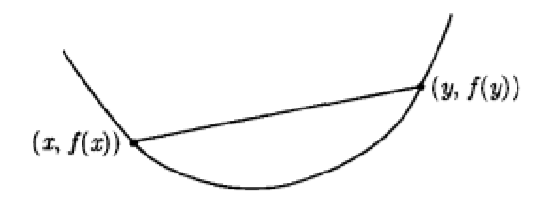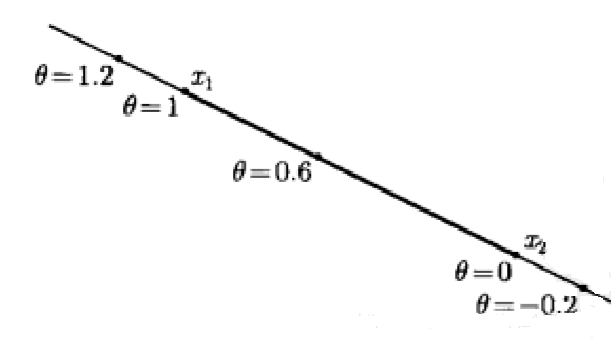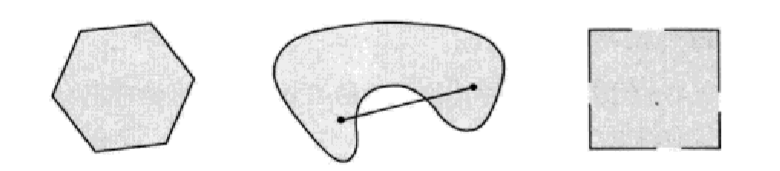• [用抽象代数讨论仿射变换和仿射空间中的坐标变换] 是重新整理的，以下是之前的内容。这里谈论的是计算机图形学中的内容，当然源自数学，但有的地方没有使用纯粹数学上的那种严格定义。线性变换抽象定义域F上的向量...
(ง •_•)ง [用抽象代数讨论仿射变换和仿射空间中的坐标变换] 是重新整理的，以下是之前的内容。

这里谈论的是计算机图形学中的内容，当然源自数学，但有的地方没有使用纯粹数学上的那种严格定义。
线性变换
抽象定义
域F上的向量空间V，任意的x、y∈V a∈F, 若 映射f: V->V满足 f(x+y)=f(x)+f(y) f(ax)=af(x) 则映射f是一个线性变换。
矩阵表示

When V are finite dimensional, a general linear transformation can be written as a matrix multiplication only after specifying a vector space basis for V. http://mathworld.wolfram.com/LinearTransformation.html

ai∈F, xi∈V, i=1,2,…,n 则 f( a1x1+a2x2+…+anxn ) = a1f(x1)+a2f(x2)+…+anf(xn)
假设(e1,e2,…,en)是向量空间V的一组基底， 则xi可以唯一地表示成：xi1e1+xi2e2+…+xinen
行向量(xi1,xi2,…,xin)的转置记作(xi1,xi2,…,xin)’ 记xi=(xi1,xi2,…,xin)’ ——（列向量形式的）坐标，以下不区分向量和它的坐标：
则 e1=(1,0,…,0)’ e2=(0,1,…,0)’ … en=(0,0,…,1)’ （xi1,xi2,…,xin∈域F，1是域F的乘法幺元。） 则 f( a1x1+a2x2+…+anxn ) = a1f(x1)+a2f(x2)+...+anf(xn) = ( f(x1),f(x2),...,f(xn) )(a1,a2,…,an)’
则 f(xi)=f( xi1e1+xi2e2+…+xinen )= (f(e1),f(e2),…,f(en))(xi1,xi2,…,xin)’ 记(f(e1),f(e2),…,f(en))=M，则

f(xi) = Mxi 其中M=(f(e1),f(e2),…,f(en)) 即线性变换由基底的变换唯一确定

仿射变换
仿射空间
域F上的向量空间V 和 非空集合A，对于任意的 p∈A v∈V，p+v ∈A，且： ①任意的 a b ∈V: (p+a)+b=p+(a+b) ②任意的q∈A，有且只有一个u∈V，使得 p+u = q A中的元素就称为点，V中的元素称为向量（自由向量）
由②可以定义“点的减法”： 既然任意的p,q∈A, 存在唯一的u: p+u=q 那么定义q-p=u
仿射变换的抽象定义
映射 f : V∪A → V∪A 满足 ①若p∈A, 则f( p)∈A ②若v∈V, 则f(v)∈V 且 任意的x、y∈V a∈域F: f(x+y)=f(x)+f(y), f(ax)=af(x) ③p∈A, v∈V, f(p+v) = f( p) + f(v) 即 q,p∈A, f(q)-f( p)=f(q-p) f 就是仿射变换。
即 f 把向量变换成向量，把点变换成点，对向量的变换是线性变换， 对点和向量的加法也有合适的体现。 可见 仿射变换 包含 线性变换。
仿射变换的矩阵表示
随便从A中找一个点，记做Q，则A中任意的点p，存在唯一的向量v∈V，使得 p = Q + v 若V是有限维度的，给V指定一组基底，从而向量v具有坐标， 这时，Q叫做原点，v的坐标称为点p的坐标；易知原点Q的坐标是零。  任意的p∈A, f( p) = f(Q+v) = f(Q) + f(v) 因为 f 是V上的线性变换，所以 f(v) = Mv (M是矩阵) 记 f(Q) 的坐标为t 所以 f( p) = t + Mv 因为 p的坐标就是v的坐标，且用记号p,v既代表点和向量，也代表它们的坐标 所以 写成 f( p) = t + Mp
所以仿射变换的形式就是（p,v既代表点和向量，也代表它们的坐标）

若 v∈V, 则 f(v) = Mv 若 p∈A, 则 f( p) = Mp + t 其中M = (f(e1),f(e2),…,f(en)), t = f(Q) 即仿射变换由基底和原点的变换唯一确定

总结一下就是只要能写成坐标，就能用矩阵表示出来。

补充
仿射坐标
引入
仿射变换对点和向量有不同的形式，想把这两种形式统一起来

f

(

p

)

=

M

p

+

t

=

M

p

+

t

⋅

1

=

(

M

t

)

(

p

1

)

f( p) = Mp+t = Mp + t·1 = (M t)\begin{pmatrix} p \\ 1 \end{pmatrix}

f

(

v

)

=

M

v

=

M

v

+

t

⋅

0

=

(

M

t

)

(

v

0

)

f(v) = Mv = Mv +t·0 = (M t)\begin{pmatrix} v \\ 0 \end{pmatrix}

定义
p,v既代表点和向量，也代表它们的坐标； 定义 点的仿射坐标为

(

p

1

)

\begin{pmatrix} p \\ 1 \end{pmatrix}

向量的仿射坐标为

(

v

0

)

\begin{pmatrix} v \\ 0 \end{pmatrix}

使用仿射坐标的矩阵表示
希望有个矩阵A,

A

(

p

1

)

=

(

f

(

p

)

1

)

A

(

v

0

)

=

(

f

(

v

)

0

)

A\begin{pmatrix} p\\1 \end{pmatrix} =\begin{pmatrix} f( p)\\1 \end{pmatrix} \qquad \qquad A\begin{pmatrix} v\\0 \end{pmatrix} =\begin{pmatrix} f(v)\\0 \end{pmatrix}

用x代表v或p 用b代表0或1

A

(

x

b

)

=

(

f

(

x

)

b

)

A\begin{pmatrix} x\\b \end{pmatrix} =\begin{pmatrix} f(x)\\b \end{pmatrix}

现在已经知道

(

M
&ThickSpace;

t

)

(

x

b

)

=

M

x

+

t

⋅

b

=

f

(

x

)

(M\;t)\begin{pmatrix} x\\b \end{pmatrix}=Mx+t\cdot b=f(x)

只需求n+1维行向量 (k_n, k)

(

k

n

,

k

)

(

x

b

)

=

b

(k_n, k)\begin{pmatrix} x\\b \end{pmatrix}=b

所以 (k_n, k) = (0, 1); 0代表n维零向量， 则

A

=

[

M

t

0

1

]

{\Large A}=\begin{bmatrix} M &amp; t\\ 0 &amp; 1 \end{bmatrix}

这样仿射变换就能统一地表示成 Aa，更高维空间中的线性变换。 其中a=

(

x

b

)

\begin{pmatrix}x\\b\end{pmatrix}

b=0或1
仿射变换矩阵的逆
假设

[

M

t

0

1

]

[

N

d

w

s

]

=

[

I

0

0

1

]

\begin{bmatrix} M &amp; t\\ 0 &amp; 1 \end{bmatrix}\begin{bmatrix} N &amp; d\\ w &amp; s \end{bmatrix}=\begin{bmatrix} I &amp; 0\\ 0 &amp; 1 \end{bmatrix}

∵

[

0

1

]

[

N

d

w

s

]

=

[

w

s

]

\because \begin{bmatrix}0 &amp;1 \end{bmatrix}\begin{bmatrix} N &amp; d\\ w &amp; s \end{bmatrix}= \begin{bmatrix}w &amp;s \end{bmatrix}

∴
&ThickSpace;

w

=

0

s

=

1

∴
&ThickSpace;

M

N

=

I

M

d

+

s

t

=

M

d

+

t

=

0

∴

[

M

t

0

1

]

−

1

=

[

M

−

1

−

M

−

1

t

0

1

]

\therefore \begin{bmatrix} M &amp; t\\ 0 &amp; 1 \end{bmatrix}^{-1}=\begin{bmatrix} M^{-1} &amp; -M^{-1}t\\ 0 &amp; 1 \end{bmatrix}

平移变换
平移变换矩阵
v*=Mv p*=Mp+t 平移变换的平移向量 为 c，则 M=I（单位矩阵），t = c 所以平移变换矩阵为

[

I

c

0

1

]

\begin{bmatrix} I &amp; c\\ 0 &amp; 1 \end{bmatrix}

平移变换矩阵的逆

[

I

−

c

0

1

]

\begin{bmatrix} I &amp; -c\\ 0 &amp; 1 \end{bmatrix}

平移与复合变换矩阵
①任意的仿射变换 都能看成 一系列变换之后 跟一个以 t 为平移向量的平移变换的复合( t 是该仿射变换的矩阵的前n行的最后一列)：

[

I

t

0

1

]

[

M

0

0

1

]

=

[

M

t

0

1

]

\begin{bmatrix} I &amp; t\\ 0 &amp; 1 \end{bmatrix}\begin{bmatrix} M &amp; 0\\ 0 &amp; 1 \end{bmatrix}=\begin{bmatrix} M &amp; t\\ 0 &amp; 1 \end{bmatrix}

②平移变换的复合（平移变换矩阵的相乘）构成交换群。

[

I

v

0

1

]

[

I

t

0

1

]

=

[

I

t

+

v

0

1

]

\begin{bmatrix} I &amp; v\\ 0 &amp; 1 \end{bmatrix}\begin{bmatrix} I &amp; t\\ 0 &amp; 1 \end{bmatrix}=\begin{bmatrix} I &amp; t+v\\ 0 &amp; 1 \end{bmatrix}

[

I

v

0

1

]

[

M

t

0

1

]

=

[

M

t

+

v

0

1

]

\begin{bmatrix} I &amp; v\\ 0 &amp; 1 \end{bmatrix}\begin{bmatrix} M &amp; t\\ 0 &amp; 1 \end{bmatrix}=\begin{bmatrix} M &amp; t+v\\ 0 &amp; 1 \end{bmatrix}


展开全文线性变换
• 仿射变换定义为一个线性变换加上平移变换。即： g(v⃗)=f(v⃗)+b⃗ g(\vec v) = f(\vec v) + \vec b g(v)=f(v)+b 仿射变换的矩阵表示 g(v⃗)=[x,y,z]⋅[f(i⃗)f(j⃗)f(k⃗)]+b⃗ g(\vec v) = [x, y, z] \cdot \begin...


仿射变换
什么是仿射变换？仿射变换的矩阵表示坐标系变换

什么是仿射变换？
仿射变换定义为一个线性变换加上平移变换。即：

g

(

v

⃗

)

=

f

(

v

⃗

)

+

b

⃗

g(\vec v) = f(\vec v) + \vec b

仿射变换的矩阵表示

g

(

v

⃗

)

=

[

x

,

y

,

z

]

⋅

[

f

(

i

⃗

)

f

(

j

⃗

)

f

(

k

⃗

)

]

+

b

⃗

g(\vec v) = [x, y, z] \cdot \begin{bmatrix} f(\vec i) \\ f(\vec j) \\ f(\vec k) \end{bmatrix} + \vec b

这里，我们要对向量

v

⃗

=

(

x

,

y

,

z

)

\vec v = (x, y, z)

转换为齐次坐标

(

x

,

y

,

z

,

w

)

(x,y,z,w)

，同样变换后的

g

(

v

⃗

)

=

(

x

′

,

y

′

,

z

′

,

w

)

g(\vec v) = (x', y', z', w)

也是齐次坐标。从而有：

g

(

v

⃗

)

=

[

x

,

y

,

z

,

w

]

⋅

[

f

(

i

⃗

)

0

f

(

j

⃗

)

0

f

(

k

⃗

)

0

0

0

]

+

[

x

,

y

,

z

,

w

]

⋅

[

0

0

0

0

0

0

b

⃗

1

]

=

[

x

,

y

,

z

,

w

]

⋅

[

f

(

i

⃗

)

0

f

(

j

⃗

)

0

f

(

k

⃗

)

0

b

⃗

1

]

\begin{aligned} g(\vec v) &= [x, y, z, w] \cdot \begin{bmatrix} f(\vec i) & 0 \\ f(\vec j) & 0 \\ f(\vec k) & 0 \\ 0 & 0 \end{bmatrix} + [x, y, z, w] \cdot \begin{bmatrix} 0 & 0 \\ 0 & 0 \\ 0 & 0 \\ \vec b & 1 \end{bmatrix} \\ &= [x, y, z, w] \cdot \begin{bmatrix} f(\vec i) & 0 \\ f(\vec j) & 0 \\ f(\vec k) & 0 \\ \vec b & 1 \end{bmatrix} \end{aligned}

对于向量而言，

w

w

分量为0，因为平移对向量不起作用，对于点而言，

w

w

分量为1。
坐标系变换
在实际运用中经常遇到一个向量或者一个点在不同坐标系下的坐标表示。先来看看向量的情形：
对于向量

v

⃗

\vec v

，它在坐标系

A

A

下的坐标为

(

x

,

y

,

z

)

(x, y, z)

，求在坐标系

B

B

下的坐标

(

x

′

,

y

′

,

z

′

)

(x', y', z')

。
实际上，无论坐标系如何变化，向量自身是不变的，那么我们有：

v

⃗

=

x

′

i

⃗

B

+

y

′

j

⃗

B

+

z

′

k

⃗

B

=

x

i

⃗

A

+

y

j

⃗

A

+

z

k

⃗

A

\vec v = x'\vec i_B + y'\vec j_B + z'\vec k_B = x\vec i_A + y\vec j_A + z\vec k_A

写成矩阵形式：

[

x

′

,

y

′

,

z

′

]

=

[

x

,

y

,

z

]

⋅

[

i

⃗

A

j

⃗

A

k

⃗

A

]

[x',y',z'] = [x,y,z] \cdot \begin{bmatrix} \vec i_A \\ \vec j_A \\ \vec k_A \end{bmatrix}

其中，

i

⃗

A

，

j

⃗

A

，

k

⃗

A

\vec i_A，\vec j_A，\vec k_A

是坐标系

A

A

的基向量在坐标系

B

B

的表示。类似地，反过来也可以得到：

[

x

,

y

,

z

]

=

[

x

′

,

y

′

,

z

′

]

⋅

[

i

⃗

B

j

⃗

B

k

⃗

B

]

[x,y,z] = [x',y',z'] \cdot \begin{bmatrix} \vec i_B \\ \vec j_B \\ \vec k_B \end{bmatrix}

其中，

i

⃗

B

，

j

⃗

B

，

k

⃗

B

\vec i_B，\vec j_B，\vec k_B

是坐标系

B

B

的基向量在坐标系

A

A

的表示。这里，可以发现一个有趣的现象：坐标系

A

A

的基向量在坐标系

B

B

的矩阵表示乘以坐标系

B

B

的基向量在坐标系

A

A

的矩阵表示得到的是单位矩阵。即：

[

i

⃗

A

j

⃗

A

k

⃗

A

]

⋅

[

i

⃗

B

j

⃗

B

k

⃗

B

]

=

I

\begin{bmatrix} \vec i_A \\ \vec j_A \\ \vec k_A \end{bmatrix} \cdot \begin{bmatrix} \vec i_B \\ \vec j_B \\ \vec k_B \end{bmatrix} = I

我们可以用三角函数的方法去证明它。
然后我们来看看点的情形。对于点

p

⃗

\vec p

，它在坐标系

A

A

下的坐标为

(

x

,

y

,

z

)

(x, y, z)

，求在坐标系

B

B

下的坐标

(

x

′

,

y

′

,

z

′

)

(x', y', z')

。容易知道结果是类似的：

p

⃗

=

x

′

i

⃗

B

+

y

′

j

⃗

B

+

z

′

k

⃗

B

+

o

⃗

B

=

x

i

⃗

A

+

y

j

⃗

A

+

z

k

⃗

A

+

o

⃗

A

\vec p = x'\vec i_B + y'\vec j_B + z'\vec k_B + \vec o_B = x\vec i_A + y\vec j_A + z\vec k_A + \vec o_A

其中

o

o

为坐标系的原点坐标。写成矩阵形式：

[

x

′

,

y

′

,

z

′

,

1

]

=

[

x

,

y

,

z

,

1

]

⋅

[

i

⃗

A

0

j

⃗

A

0

k

⃗

A

0

o

⃗

A

1

]

[x',y',z', 1] = [x,y,z, 1] \cdot \begin{bmatrix} \vec i_A & 0 \\ \vec j_A & 0 \\ \vec k_A & 0 \\ \vec o_A & 1 \end{bmatrix}

其中，

i

⃗

A

，

j

⃗

A

，

k

⃗

A

,

o

⃗

A

\vec i_A，\vec j_A，\vec k_A, \vec o_A

是坐标系

A

A

的基向量和原点在坐标系

B

B

的表示。
展开全文线性代数
• 函数f:Rn→Rf:\mathbf{R}^{n} \rightarrow \mathbf{R}f:Rn→R是凸的，如果fff在定义域(domdomdom)上是凸集，且对于任意x,y∈domfx,y∈\mathbf{dom}fx,y∈domf和任意0⩽θ⩽10⩽θ⩽10⩽θ⩽1，有 f(θx+(1−θ)y)⩽...
凸优化（Stephen Boyd)中自学部分
凸函数定义
函数

f

:

R

n

→

R

f:\mathbf{R}^{n} \rightarrow \mathbf{R}

是凸的，如果

f

f

在定义域(

d

o

m

dom

)上是凸集，且对于任意

x

,

y

∈

d

o

m

f

x,y∈\mathbf{dom}f

和任意

0

⩽

θ

⩽

1

0⩽θ⩽1

，有

f

(

θ

x

+

(

1

−

θ

)

y

)

⩽

θ

f

(

x

)

+

(

1

−

θ

)

f

(

y

)

f(\theta x+(1-\theta) y) \leqslant \theta f(x)+(1-\theta) f(y)

。凸函数示意图，图中点

(

x

,

f

(

x

)

)

(x,f(x))

到

(

y

,

f

(

y

)

)

(y,f(y))

之间的线段都在函数

f

f

的图像上方。函数是凸函数，当且仅当其在与其定义域相交的任何直线上都是凸的。
为了理解，补充直线和线段的知识：
直线和线段
设

x

1

≠

x

2

x_{1} \neq x_{2}

为

R

n

R^n

空间中的两个点，则

y

=

θ

x

1

+

(

1

−

θ

)

x

2

,

θ

∈

R

y=\theta x_{1}+(1-\theta) x_{2}, \theta \in \mathbf{R}

组成一条穿越

x

1

x_{1}

和

x

2

x_{2}

的直线，参数

θ

\theta

的值在0和1之间变动。这里的

x

1

x_{1}

和

x

2

x_{2}

可以类比上述提到的

(

x

,

f

(

x

)

)

(x,f(x))

和

(

y

,

f

(

y

)

)

(y,f(y))

。个人理解：一般我们在实际应用中，用到的凸函数偏多，凹函数可以取负号变换为凸函数。
仿射集合
判断集合

C

∈

R

n

C∈R^n

为仿射集合，则对于任意的

x

1

，

x

2

∈

C

x_{1} ，x_{2}∈C

及

θ

∈

R

\theta∈R

，有

θ

x

1

+

(

1

−

θ

)

x

2

∈

C

\theta x_{1}+(1-\theta) x_{2} \in C

，也就是

C

C

包含了

C

C

中任意两点的系数之和为1的线性组合。此概念拓展到多个点也适用。
例子： 线性方程组的解集

C

=

{

x

∣

A

x

=

b

}

C=\{x | A x=b\}

是一个仿射集合，其中

A

∈

R

m

×

n

A \in \mathbf{R}^{m \times n}

,

B

∈

R

m

B \in \mathbf{R}^{m}

.
证明：可设

x

1

，

x

2

∈

C

x_{1} ，x_{2}∈C

，有

A

x

1

=

b

,

A

x

2

=

b

A x_{1}=b, A x_{2}=b

，对于任意

θ

\theta

，

A

(

θ

x

1

+

(

1

−

θ

)

x

2

)

=

θ

A

x

1

+

(

1

−

θ

)

A

x

2

=

θ

b

+

(

1

−

θ

)

b

=

b

A\left(\theta x_{1}+(1-\theta) x_{2}\right)=\theta A x_{1}+(1-\theta) A x_{2}=\theta b+(1-\theta) b=b

，说明任意的仿射组合

θ

x

1

+

(

1

−

θ

)

x

2

\theta x_{1}+(1-\theta) x_{2}

也在

C

C

中。
凸集
注意：这里的凸集和凸函数不是一个概念。 判断某个集合是否为凸集，看集合中任意两点之间的线段是否在集合中。举个例子：上图中，（1）包含其边界的六边形是凸的。（2）肾形集合不是凸的，因为图中所示集合中两点间的线段不为集合所包含。（3）仅包含部分边界的正方形不是凸的。
仿射函数
白话理解就是仿射函数是一个线性函数和一个常数的和，也就是具有

f

(

x

)

=

A

x

+

b

f(x)=A x+b

的形式，其中

A

∈

R

m

×

n

A \in \mathbf{R}^{m \times n}

,

B

∈

R

m

B \in \mathbf{R}^{m}

。
假设

S

⊆

R

n

S \subseteq \mathbf{R}^{n}

是凸的（即

S

S

为凸集），并且

f

:

R

n

→

R

m

f: \mathbf{R}^{n} \rightarrow \mathbf{R}^{m}

是仿射函数，则

S

S

在

f

f

下的象

f

(

S

)

=

{

f

(

x

)

∣

x

∈

S

}

f(S)=\{f(x) | x \in S\}

是凸的。
展开全文算法
• ## 仿射空间

千次阅读 2019-09-27 14:47:16
向量空间（也叫线性空间）我们是很熟悉的，那么可以借助向量空间给仿射空间一个直观的定义仿射空间就是没有原点的向量空间。 向量空间没有了原点，会有什么样的影响？向量是基于原点的，向量有两个元素-大小、...
直观理解仿射空间
向量空间（也叫线性空间）我们是很熟悉的，那么可以借助向量空间给仿射空间一个直观的定义：仿射空间就是没有原点的向量空间。
向量空间没有了原点，会有什么样的影响？向量是基于原点的，向量有两个元素-大小、方向，大小任意给出两个点就可以衡量，但没有了原点，我们是无法用一个统一的标准来衡量方向的，也正因如此，仿射空间包含点集而不单纯是向量集。
更进一步，没有了统一标准的向量，那么向量与向量之间的关系也发生了变化，向量空间的2向量之间可以有"+"运算；而在仿射空间中，点与点之间没有加法运算，为了衡量从一个点到另一个点之间的关系，点x需要加上某个向量v来的到点y，这个运算过程叫做translation。
以上，而我们知道定义仿射空间的两个要素：1. 没有原点的点集。2. 点与点之间的translation运算（或者说向量V）

正式定义

一个仿射空间就是一个点集X和一个向量集V（X和V维度相同），它满足以下公理：  （1）对所有 x∈X 和 u,v∈V ，满足 x+(u+v)=(x+u)+v 。即translation复合后作用于x等价于两个translation分别作用于x  （2）对所有 x∈X ， x+0=x ，即x加上0向量等于其自身  （3）对所有 x∈X ，如果存在 v∈V ，使得 x+v=x ，则 v=0  （4）对所有的 x，y∈X ，存在一个 v∈V ，使得 y=x+v

仿射变换
相对于仿射空间，仿射变换就比较容易理解了，我们知道线性变换的几何意义是图像的旋转和变形，而仿射变换的几何意义是旋转和变性再加上位移，所以仿射变换也可以表示为线性变换再加上位移。用矩阵表示的话，线性变换是 y = Ax，而仿射变换是    y = Ax + b。

参考文献：
 Affine space
 仿射空间（affine space），仿射组合（affine combination）
展开全文• 仿射组合（Affine Combination） 对于数域为 FF\mathbb F 的线性空间 VVV 中的任意 nnn 个向量 a1,⋯,an,a1,⋯,an,a_1, \cdots, a_n, 称向量 β=∑i=1nxiαi,β=∑i=1nxiαi,\beta = \sum \limits_{i = 1} ^{n} x ...
• 语言：python3 实验目的： 通过编程实现代换密码算法——仿射密码和简单置换密码算法，加深对古典密码体制的了解，为深入学习密码学奠定基础。 实验内容： ... 仿射密码加密解密模块 @author WQ @.python
• 在几何上定义为两个向量空间之间的一个仿射变换或者仿射映射（来自拉丁语，affinis，“和。..相关”）由一个线性变换接上一个平移组成。
• 前言：摘抄自wiki的关于仿射变换的定义仿射变换，又称仿射映射，是指在几何中，一个向量空间进行一次线性变换并接上一个平移，变换为另一个向量空间。一个对向量平移，与旋转放大缩小的仿射映射为 【1】1、移位...
• ## MATLAB 仿射变换

万次阅读 2017-06-04 11:34:35
如下图，matlab中通过定义一个3×3的矩阵实现仿射变换（平移、缩放、旋转等）。注意到，变换矩阵的第三列恒为【0,0,1】，实际上，确定一个仿射变换只需要一个2×3的变换矩阵即可。matlab 仿射变换主要有三种常用形式...matlab
• ## 仿射函数

千次阅读 2018-06-05 00:22:33
仿射函数编辑词条仿射函数即由由1阶多项式构成的函数，一般形式为 f (x) = A x + b，这里，A 是一个 m×k 矩阵，x 和 b 都是一个 m 向量，实际上反映了一种从 k 维到 m 维的空间映射关系。设f是一个矢性（值）函数，...
• 在几何上定义为两个向量空间之间的一个仿射变换或者仿射映射（来自拉丁语，affinis，“和。..相关”）由一个线性变换接上一个平移组成。 目录 1原理 2示例 3相关例子 1原理编辑 在...
• 3. 定义4. 常用的转换矩阵5.实际应用5.1 栗子15.2 栗子2 1. 引用 opencv学习(三十五)之仿射变换warpAffine 2. 什么是仿射变换？ 3. 定义 向量空间中进行一次线性变换(乘以一个矩阵A)再加上一个平移(加上一个向量B)...
• 仿射变换和透视变换和图像坐标系、相机坐标系和世界坐标系的定义开篇仿射变换透视变换图像坐标系、相机坐标系和世界坐标系三维的点投影在二维平面上坐标系和坐标变换公式合体Zhang方法 开篇 本文主要介绍计算机视觉...
• 文章目录定义一、性质二、例程 定义 一个任意的仿射变换都能表示为 乘以一个矩阵 (线性变换) 接着再 加上一个向量 (平移). 综上所述, 我们能够用仿射变换来表示:： 旋转 (线性变换) 平移 (向量加) 缩放操作 (线性...
• 沿一个公共点(投影中心) 的射线的投影定义了从一张平面到另一张平面上的映射 (参考射影平面的定义). 在透视影像下形状会失真. 例如实际中景物平面上的平行线在图像上一般地不平行并会聚到一个有限点. 由于平面......